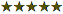Circle Imprint Beverage Napkins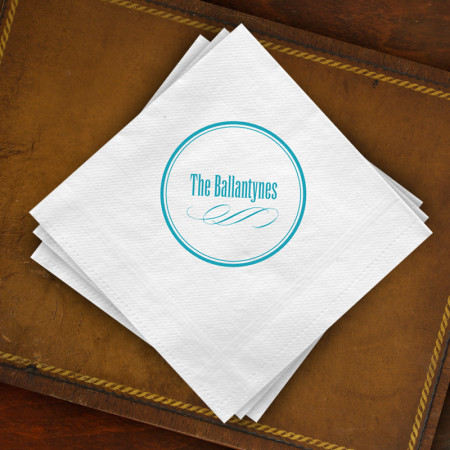Double click on above image to view full picture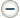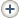Circle Imprint Beverage Napkins

Price per Box: \$?.??/Box , 1 Box = 50 Bev. Napkins

 Quantity: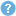0 = \$0.00 1 = \$37.00 2 = \$45.00 3 = \$65.00 4 = \$80.00 5 = \$95.00 6 = \$110.00 7 = \$125.00 8 = \$140.00 9 = \$155.00 10 = \$170.00 11 = \$185.00 12 = \$200.00 13 = \$215.00 14 = \$230.00 15 = \$245.00 16 = \$260.00 17 = \$275.00 18 = \$290.00 19 = \$305.00 20 = \$320.00 21 = \$335.00 22 = \$350.00 23 = \$365.00 24 = \$380.00 +MORE Printing Process:Letterpress
Item # 5058 Napkins Letterpress 5" x 5"

Personalize
Description

Details

Add instant sophistication with the classic simplicity of these stylishly designed White napkins. A circular border surrounds your personalization in the type style shown.

• Type Style: As Shown
• Set includes 50 napkins
• Reviews

Be the first to review this product

You're reviewing:

Circle Imprint Beverage Napkins

How do you rate this product? *

 1 star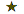2 stars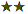3 stars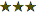4 stars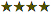5 stars